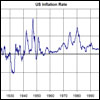14.01SC | Fall 2011 | Undergraduate

# Principles of Microeconomics

Unit 6: Topics in Intermediate Microeconomics

## Capital Supply and Markets I

« Previous | Next »

### Session OverviewDuring most of our discussion of consumer decisions and the production decisions of firms, we have focused on only labor and the decisions that individuals make about whether or not to work. We know from our study of production functions that firms also use capital to produce output. Where does capital come from? Not surprisingly, it is the result of another consumer decision, the decision of whether or not to save. This lecture analyzes the decisions consumers and firms make in the capital market. Inflation rates change the value of a dollar from year to year. This image is in the public domain. Source: Wikipedia.

Keywords: Capital markets; intertemporal choice; present value; future value; inflation; real interest rate; compounding.

### Session Activities

Before watching the lecture video, read the course textbook for an introduction to the material covered in this session:

• [R&T] Chapter 13, “Interest Rates and the Markets for Capital and Natural Resources.”
• [Perloff] Chapter 16, “Interest Rates, Investments, and Capital Markets.” (optional)

### Check Yourself

#### Concept Quiz

This concept quiz covers key vocabulary terms and also tests your intuitive understanding of the material covered in this session. Complete this quiz before moving on to the next session to make sure you understand the concepts required to solve the mathematical and graphical problems that are the basis of this course.

###### Question 1

What is the price of consuming a certain good this year as opposed to next year? Note that i denotes the interest rate, and w denotes the wage.

1 + i check
The price depends on how much you are saving. close
i close
w close

The correct answer is that the price of consuming a certain good this year as opposed to next year is 1 + i. If you decide not to consume and instead save a dollar, you will receive 1 + i back in exchange for that dollar next year. Thus the price of consuming this year as opposed to consuming exactly the same good next year is 1 + i.

###### Question 2

When the interest rate increases, what is the impact on current consumption?

It decreases. close
It increases. close
It remains the same. close
The effect is ambiguous. check

When the interest rate increases, there are two effects on current consumption. The first is the substitution effect: current consumption is now more expensive relative to future consumption, so one should consume less. The second is the income effect: as a result of earning a higher interest rate on their savings, consumers are now richer, and may want to consume more. The overall effect is ambiguous.

###### Question 3

Assume you have the option to choose between receiving a given amount of money M 5 years from now or 10 years from now, and the interest rate is positive. Which option has the higher present value?

It depends on the level of the interest rate. close
Receive the money 10 years from now. close
Receive the money 5 years from now. check
Receiving M always has the same present value, independent of the timing. close

If the interest rate is positive, then it is always preferable to receive the same amount of money sooner rather than later.

###### Question 4

What is the definition of the real interest rate?

None of these. close
The nominal interest rate minus inflation. check
The nominal interest rate plus inflation. close
The ratio of the nominal interest rate to the wage. close

When you save money, you put dollars in the bank today in exchange for dollars in the bank tomorrow. However, a dollar may not be worth the same amount tomorrow in purchasing power terms. In particular, if the prices of the goods you want to consume are increasing very rapidly, then a dollar tomorrow may have very little value. The nominal interest rate thus captures the rate of return you earn on your savings in terms of purchasing power, and it is calculated by subtracting the rate of inflation from the nominal interest rate.

« Previous | Next »

## Course Info

Fall 2011
##### Learning Resource Types
Lecture Videos
Recitation Videos
Problem Sets with Solutions
Exams with Solutions
Exams
Lecture Notes
Exam Materials
Problem Sets